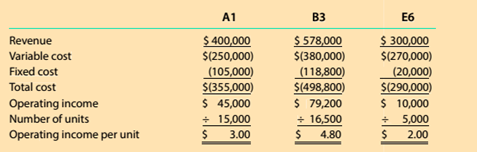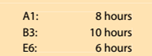Chapter 12, Problem 12.1MBA

Chapter
Section
Textbook Problem

Contribution margin per constraint Zion Metals Inc. has three grades of metal product. Al, B V and E6. Financial data for the three grades are as follows:Zion Metals' operations require all three grades to be melted in a furnace before being formed. The furnace runs 24 hours a day. 7 days a week, and is a production constraint. The furnace hours required per unit of each product are as follows:The Marketing Department is considering a new marketing and sales campaign. Which product should be emphasized in the marketing and sales campaign in order to maximize profitability?

To determine

Concept Introduction:

Cost Volume Profit (CVP) Analysis:

The Cost Volume Profit analysis is the analysis of the relation between cost, volume, and profit of a product. It analyzes the cost and profits at the different level of production, in order to determine the breakeven point and required the level of sales to earn the desired profit.

Contribution margin means the margin that is left with the company after recovering variable cost out of revenue earned by selling smart phones.

The formula for contribution margin is as follows:

Contribution margin = Sales - Variable cost.

Similarly contribution margin ratio = Contribution/sales

To Identify:

The Product to be emphasized for marketing and sales

Explanation

The analysis is done on the basis of contribution margin per hour provided by each product as follows:

 Product A1 B3 E6 Revenue (A) \$ 400,000 \$ 578,000 300000 Variable Cost (B) \$ 250,000 \$ 380,000 270000 Contribution Margin (C) = (A-B) \$ 150,000 \$ 198,0...

Still sussing out bartleby?

Check out a sample textbook solution.

See a sample solution

The Solution to Your Study Problems

Bartleby provides explanations to thousands of textbook problems written by our experts, many with advanced degrees!

Get Started

Find more solutions based on key concepts